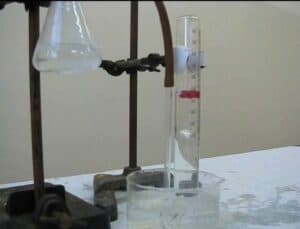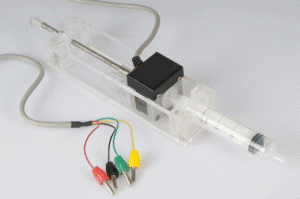## Chemical calculationsIn this activity students study the reaction between magnesium and hydrochloric acid using a pre-recorded video. The reaction equation shows that a gas is produced. By measuring the amount of gas that is produced during a certain amount of time, the reaction rate can be determined. Students follow the rate of gas production by following the position of floating plastic balls in the collecting cylinder. By using the correct scaling, the moment of the balls can be correlated to a certain volume of gas. This scaling has already been prepared so that students can focus on the chemical aspects of this experiment.In this activity students carry out a titration to determine the concentration of an acidic solution. The equivalence point can be determined from the titration curve. Compared to a “regular” titration, the use of an automated step motor burette adds an extra dimension to the experiment, allowing for addition of the base using constant speed. This experiment focusses on setting up the experiment and analysing the results.